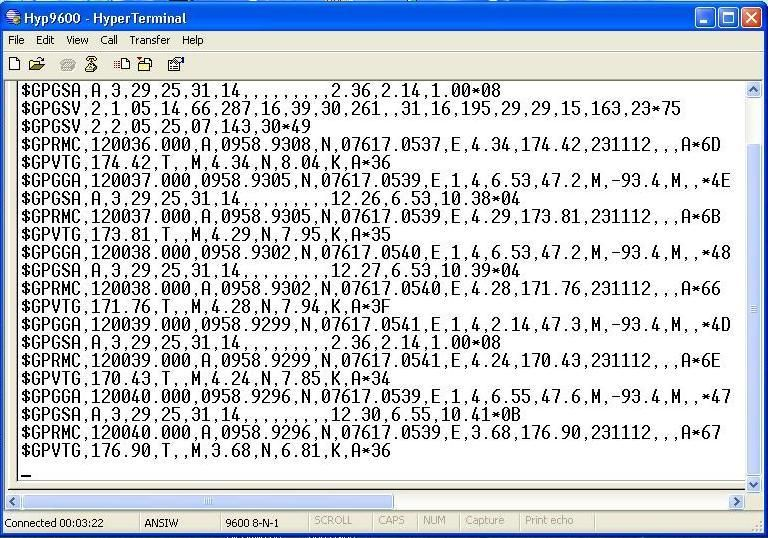# Calculate total distance travel by vehicle using GPS

## Recommended Posts

Hi guys,

I'm using L80 quectel GPS module. Which sends the NMEA protocol data string which has latitude, longitude, UTC time, knots, etc. We are going to use this GPS module in a vehicle. We have to calculate the distance travel by the vehicle using GPS. We have to show the total distance travel by the vehicle like an odometer. Is it possible to calculate total distance travelled by vehicles using "knots" value from a GPS? If it is possible, then how to calculate?

Thanks..

Edited by ms123
##### Share on other sites

You could use NavTrek charts and a marine GPS.

##### Share on other sites

Knots are a velocity.

I think if you record how long that velocity is maintained, multiply that time by the velocity and then add it to Total Distance, that could work.

##### Share on other sites

Hi Endy0816,

Thanks for the reply. The image i've attached shows the NMEA protocol string. The string data starts with $GPRMC has knots value. for example$GPRMC,123519,A,4807.038,N,01131.000,E,022.4,084.4,230394,003.1,W*6A

Where:
RMC -Recommended Minimum sentence C
123519 -Fix taken at 12:35:19 UTC
A -Status A=active or V=Void.
4807.038,N - Latitude 48 deg 07.038' N
01131.000,E - Longitude 11 deg 31.000' E
022.4 - Speed over the ground in knots
084.4 - Track angle in degrees True
230394 - Date - 23rd of March 1994
003.1,W - Magnetic Variation
*6A - The checksum data, always begins with *the image i've attached has knots 4.34, and next GPRMC data string has 4, next has 4.29, 4 and so on...

Here is that i have to calculate difference between two knots and time, then multiply the two values? for example 4.34 - 4= 0.34 knot and time 5 sec - 8sec = 3 sec, so the distance is 0.34*3=1.02 .? Is my calculation is correct?

##### Share on other sites

How accurate do you think that speed is? Do you understand how it's calculated by the GPS module?

Is it really 1994? Or in your screenshot 2012...

##### Share on other sites

Hi Klaynos,

Thanks for the reply. I dont know how is calculating in GPS?

##### Share on other sites

A GPS receiver only knows time and position. It then calculates speed from that, depending on the specific receiver will depend how often it does that calculation. If the location is not very accurate what will happen to the speed even if the box is stationary?

## Create an account

Register a new account